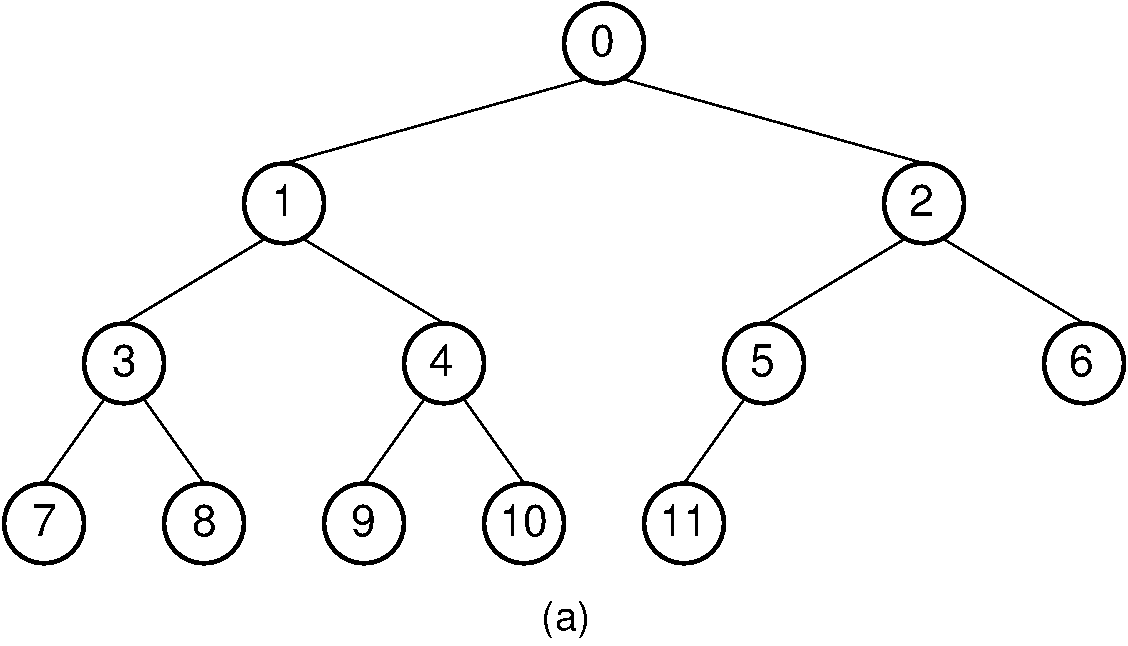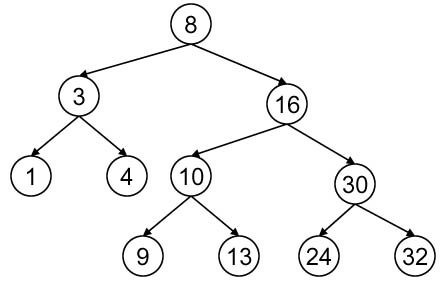×

Search anything:

# Odd even level difference in a binary tree

#### Problems on Binary Tree AlgorithmsGet this book -> Problems on Array: For Interviews and Competitive Programming

In this article, I will be discussing about the problem "Children sum parent in a Binary Tree" in detail.

Contents:

• Introduction
• Optimized approach
• Implementation
• Code Explanation
• Time and Space Complexity Analysis

## Introduction:

In this problem at OpenGenus, we are going to calculate the difference between the the sum of node values at odd levels and the sum of node values at the even levels. we will consider the root node as the first level and its left and right child as the second level and so on.

Example 1:In this example, we can see that the sum of values of the nodes at odd level are: 0+3+4+5+6 = 18 and the sum of values of the nodes at even level are:
1+2+7+8+9+10+11 = 48. so, the difference between the the sum of node values at odd levels and the sum of node values at the even levels will be: (18-48) = -30.

Example 2:In this example, we can see that the sum of values of the nodes at odd level are: 8+1+4+10+30 = 53 and the sum of values of the nodes at even level are:
3+16+9+13+24+32 = 97. so, the difference between the the sum of node values at odd levels and the sum of node values at the even levels will be: (53-97) = -44.

## Optimized Approach:

In the optimized approach, we will traverse the given binary tree in a breadth first search manner or level order traversal using queue data structure. For each level of traversal we will add all the values of a particular level and then if that current level is odd or even and accordingly we will store that sum value. After traversing the whole tree we will return the difference between the the sum of node values at odd levels and the sum of node values at the even levels.

## Implementation:

``````import java.util.*;
class Node{
int data;
Node left;
Node right;
Node(int data){
this.data = data;
left=null;
right=null;
}
}
class Solution
{
public static int getLevelDiff(Node root)
{
int sum1 = 0;
int sum2 = 0;
int x = 1;

while(q.isEmpty()==false){
int count = q.size();
for(int i = 0; i<count;i++){
Node curr = q.poll();
if(x%2!=0){
sum1+=curr.data;
}
else{
sum2+=curr.data;
}
if(curr.left!=null){
}
if(curr.right!=null){
}

}
x++;
}
return (sum1-sum2);
}
public static void main(String args[])
{
Node root = new Node(5);
root.left = new Node(2);
root.right = new Node(6);
root.left.left = new Node(1);
root.left.right = new Node(4);
root.left.right.left = new Node(3);
root.right.right = new Node(8);
root.right.right.right = new Node(9);
root.right.right.left = new Node(7);

System.out.println("difference between sums is " +
getLevelDiff(root));
}
}
``````

## Code Explanation:

1. We are creating a function getLevelDiff() and pass the root node as a parameter in this.
2. This function will return the difference between the the sum of node values at odd levels and the sum of node values at the even levels.
3. If you don't know how level order traversal of a binary tree works using a queue data structure, first you can go through this https://iq.opengenus.org/level-order-traversal-binary-tree/.
4. In level order traversal, if we are traversing the last node of a particular level then all the node of its next level must have been added to the queue till then and all the nodes of that level must been removed from the queue.
5. So we will be creating a variable count to get the total count of nodes at a particular level and a variable x to get the information about the current level and will be incrementing x after we reach the last node of a particular level.
6. If the value of x is odd then we will store the value of that node in sum1 variable and if the value of x is even then we will store the value of x in sum2 variable.
7. If the queue becomes empty, then we have traversed the whole binary tree. Therefore, we will return the value (sum1-sum2).

## Time and Space Complexity Analysis:

The time complexity of the above solution will be O(N),where N is total number of nodes in the binary tree. As in the above approach we are doing complete traversal of the binary tree, so the overall complexity of the above solution will be O(N).

The space complexity of the above solution will be O(N), where N is total number of nodes in the binary tree. Since we are creating a queue data structure and at a time the maximum nodes that the queue will store is the all nodes of a particular level which will be maximum width of the binary tree. The maximum width of binary tree can be N/2 if it is complete binary tree, so the overall space complexity of the above solution will be O(N).

With this article at OpenGenus, you must have the complete idea of solving this problem "Odd even level difference in a binary tree".

#### Uddeshya Raj

Uddeshya Raj is pursuing B. Tech in Computer Science from Chandigarh University. He is an Algorithm and Data Structure Developer, Intern at OpenGenus.